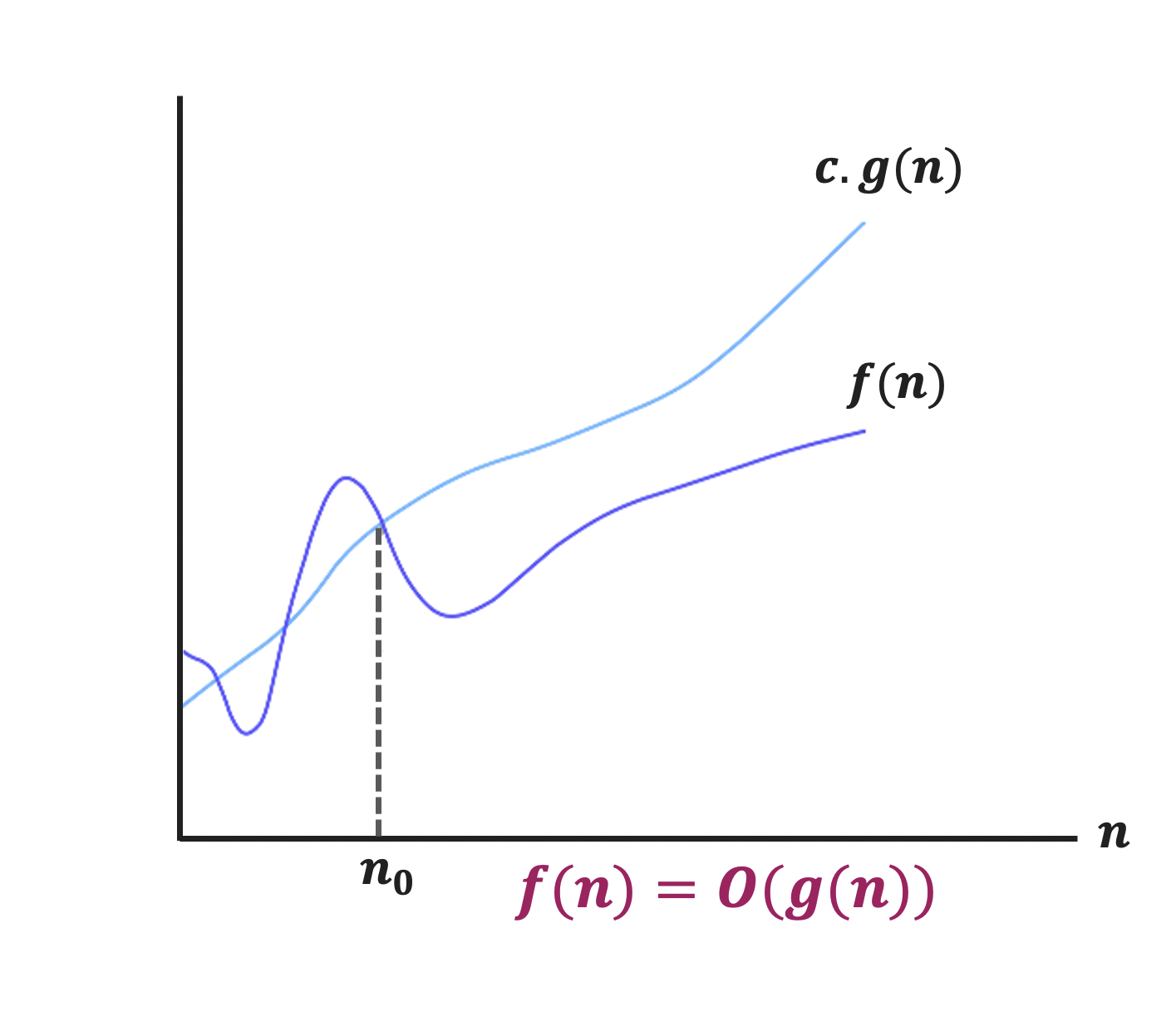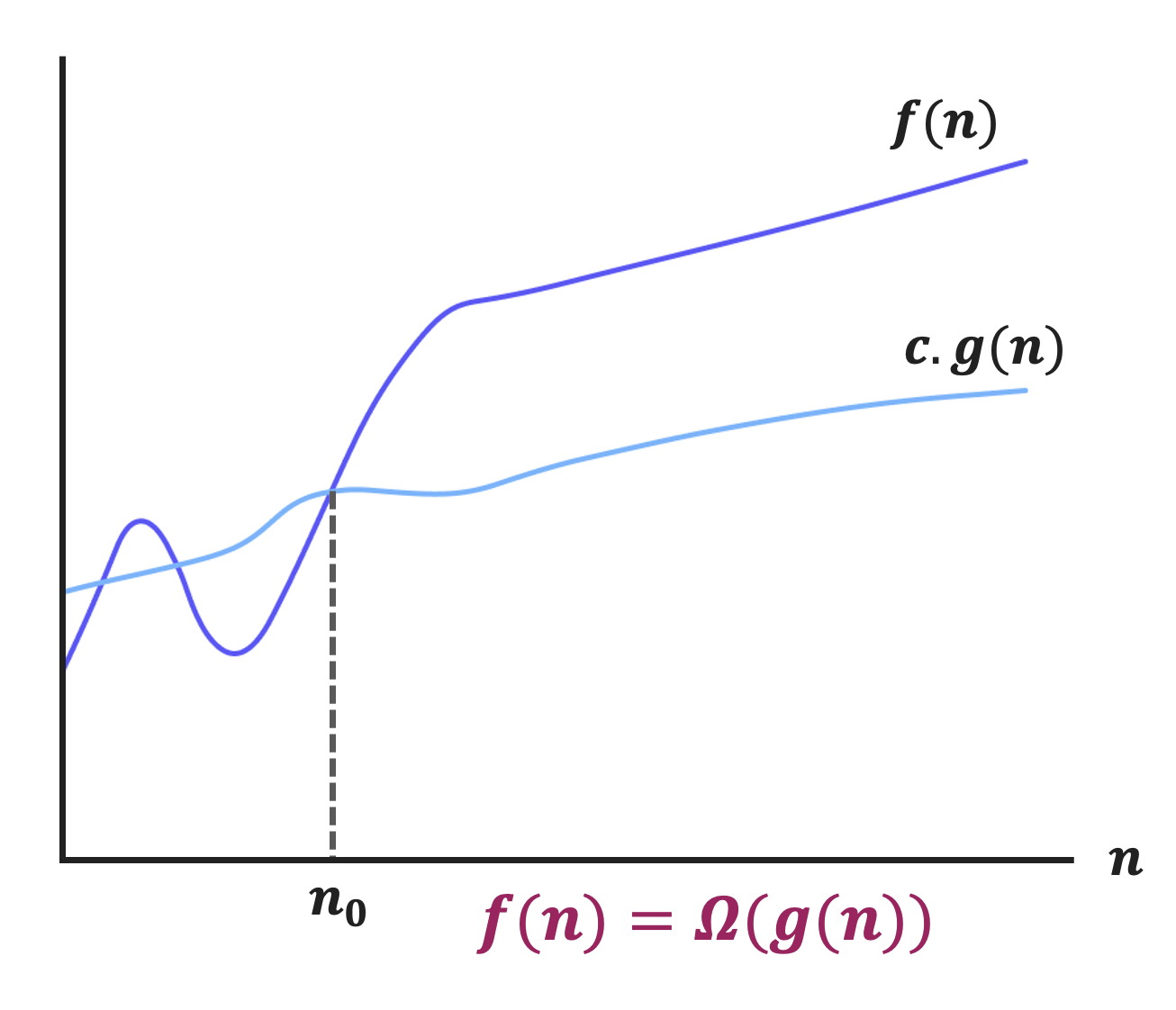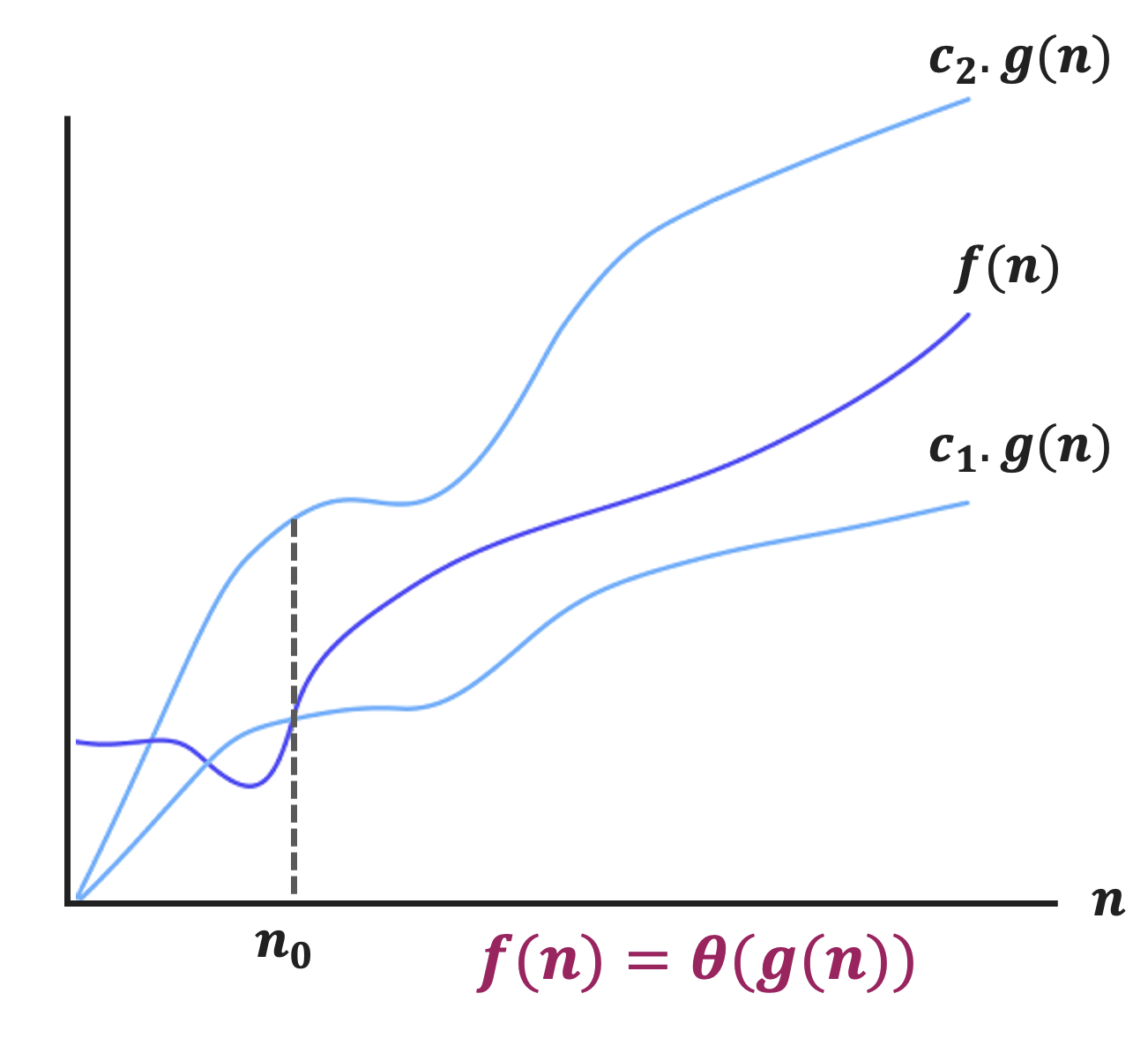Engineer bro!

Fri Apr 29 2022 (5 months ago)

Engineer by mistake!

I am a software engineer by passion

# Asymptotic analysis

The asymptotic analysis defines the mathematical foundation of an algorithm's run time performance. If there is no input to an algorithm then the algorithm will always work at a constant time. Asymptotic analysis is the running time of any process or algorithm in mathematical terms.

As we have seen in analysing-the-algorithm article, there are two types of analysis:

1. Empirical analysis - Run the Algorithm and measure how much processor time and storage are needed.

2. Theoretical analysis - Mathematically computing how much time and space are needed as a function of input size.

We have also seen that empirical analysis is not feasible as you will always have to buy the different hardware and software then run the algorithm and record the running time and storage taken by the algorithm.

So, the process of buying new hardware, and running the algorithm using different software is a tedious task.

The running time of an algorithm depends on two factors:

1. Input size

2. Nature of input

Generally, the running time grows for the algorithm along with input size, for example, sorting 100 numbers will take less time than sorting $1{0}^{10}10^\left\{10\right\}$ numbers. So, the running time of the algorithm is usually measured as the function of input size.

Instead of measuring the actual time required in executing each statement in the code, we consider how many times each statement is executed. So, the theoretical complexity of the algorithm is measured in terms of the number of steps/primitive operations performed.

## Problem & Instance

Let's see what is a problem and its instance.

### Instance

The instance of the problem consists of the input needed to compute the solution to the problem.

For example:

• Problem :- Multiply two numbers 8 and 9.

• Instance :- 8 & 9

### Instance size

Any integer(generally n) that in some way measures the number of the component in an instance.

For example:

• Sorting problem - Instance size will be the number of elements to be sorted

• Graph problem - Instance size will be the number of nodes/edges in the graph.

A linear search or sequential search is a method for finding an element within a list. It sequentially checks each element of the list until a match is found or the whole list has been searched.

Consider the below array of numbers, you need to find 41 among them.To do so, you need to go through all the positions of the array one by one. Stop the iteration once you found 41.Algorithm

function linearSearch(array,toFind){
for(var i=0; i<array.length; i++){
if(array[i] == toFind){
// return the position of the found number
return i;
}
}

return -1;
}


## Cases of algorithmic complexity

There are three cases of algorithmic complexity:• Best Case - Minimum comparison is required

• Average Case - Average number of comparisons is required

• Worst-Case - Maximum number of comparisons is required.

Best Case Average Case Worst-Case
Resource uses is minimum Resource uses is average Resource uses is maximum
Algorithm's behaviour under optimal condition Algorithm's behaviour under random condition Algorithm behaviour under worst condition
Minimum number of steps required Average number of steps required Maximum number of steps required
Lower bound on running time Average bound on running time Upper bound in running time
Generally do not occur in real Average and worst-case performance are mostly used in real life

Consider the below array of numbers.

$\left[45,78.12,90\right]\left[45,78.12,90\right]$

The best-case for linear search on the above array will be finding the number 45. As it is at the first position of the array, so it requires a single comparison.

The average-case for linear search on the above array will be finding any number between 45 and 90.

The worst-case for linear search on the above array will be finding any 90, as it requires the maximum number of comparisons.## What exactly Asymptotic analysis is?

Computing the running time of the Algorithm's operations in mathematical units of computation and defining the mathematical formula of its run time performance is referred to as Asymptotic analysis.

An algorithm may not have the same performance for different types of inputs, with the increase in the input size or nature will change the overall performance of the algorithm. As we have seen in the case of the linear search, the running time totally depends on the input given to the algorithm.

Asymptotic analysis accomplishes the study of change in performance of the algorithm with the change in the order of the input size. Using Asymptotic analysis, we can very well define the best case, average case and worst case of the algorithm.

There are three main mathematical notation is used in Asymptotic analysis:These are also known as the Algorithm's growth rate, let's see them one by one

## 1. Big Oh notation - Upper bound

The notation $O\left(n\right)O\left(n\right)$ is the formal way to express the upper bound of an Algorithm's running time. It measures the worst-case time complexity or the longest amount of time an algorithm can possibly take to complete.$O\left(g\left(n\right)\right)O\left(g\left(n\right)\right)$ = $f\left(n\right)f\left(n\right)$ : there exists some positive constants $cc$ and ${n}_{0}n_0$ such that $0<=f\left(n\right)<=cg\left(n\right)0<=f\left(n\right) <= cg\left(n\right)$ for all ${n}_{0}<=nn_0 <= n$.

• $g\left(n\right)g\left(n\right)$ is an asymptotically upper bound for $𝑓\left(𝑛\right)𝑓\left(𝑛\right)$.

• An upper bound $𝑔\left(𝑛\right)𝑔\left(𝑛\right)$ of an algorithm defines the maximum time required, we can always solve the problem in at most $𝑔\left(𝑛\right)𝑔\left(𝑛\right)$ time.

• Time taken by a known algorithm to solve a problem with worse case input gives the upper bound.

$nn$ $f\left(n\right)={n}^{2}f\left(n\right)=n^2$ $g\left(n\right)={2}^{n}g\left(n\right) = 2^n$
1 1 2 $f\left(n\right)
2 4 4 $f\left(n\right)=g\left(n\right)f\left(n\right)=g\left(n\right)$
3 9 8 $f\left(n\right)>g\left(n\right)f\left(n\right)>g\left(n\right)$
4 16 16 $f\left(n\right)=g\left(n\right)f\left(n\right)=g\left(n\right)$
5 25 32 $f\left(n\right)
6 36 64 $f\left(n\right)

## 2. Omega notation - Lower bound

Big Omega notation (Ω ) is used to define the lower bound of any algorithm or we can say the best case of any algorithm. This always indicates the minimum time required for any algorithm for all input values, therefore the best case of any algorithm. When a time complexity for any algorithm is represented in the form of big-Ω, it means that the algorithm will take at least this much time to complete it's execution. It can definitely take more time than this too.$\mathrm{\Omega }\left(g\left(n\right)\right)\Omega \left(g\left(n\right)\right)$ = { $f\left(n\right)f\left(n\right)$:There exists a positive constants $cc$ and ${n}_{0}n_0$ such that $0<=cg\left(n\right)<=f\left(n\right)0 <= cg\left(n\right) <= f\left(n\right)$ for all ${n}_{0}<=nn_0 <= n$ }

• $𝑔\left(𝑛\right)𝑔\left(𝑛\right)$ is an asymptotically lower bound for $𝑓\left(𝑛\right)𝑓\left(𝑛\right)$.

• $𝑓\left(𝑛\right)=\mathrm{\Omega }\left(𝑔\left(𝑛\right)\right)𝑓\left(𝑛\right)= \Omega \left(𝑔\left(𝑛\right)\right)$ implies: $𝒇\left(𝒏\right)\ge 𝒄\mathrm{.}𝒈\left(𝒏\right)𝒇\left(𝒏\right) \ge 𝒄.𝒈\left(𝒏\right)$

• A lower bound $𝑔\left(𝑛\right)𝑔\left(𝑛\right)$ of an algorithm defines the minimum time required, it is not possible to have any other algorithm (for the same problem) whose time complexity is less than $𝑔\left(𝑛\right)𝑔\left(𝑛\right)$ for random input.

$nn$ $g\left(n\right)={2}^{n}g\left(n\right) = 2^n$ $f\left(n\right)={n}^{2}f\left(n\right) = n^2$
1 2 1 $f\left(n\right)>g\left(n\right)f\left(n\right) > g\left(n\right)$
2 4 4 $f\left(n\right)=g\left(n\right)f\left(n\right) = g\left(n\right)$
3 8 9 $f\left(n\right)
4 16 16 $f\left(n\right)=g\left(n\right)f\left(n\right) = g\left(n\right)$
5 32 25 $f\left(n\right)>g\left(n\right)f\left(n\right) > g\left(n\right)$
6 64 36 $f\left(n\right)>g\left(n\right)f\left(n\right) > g\left(n\right)$

## 3. Theta notation - same order

The notation $\theta \left(n\right)\theta \left(n\right)$ is the formal way to enclose both the lower bound and the upper bound of an algorithm's running time. Since it represents the upper and the lower bound of the running time of an algorithm, it is used for analyzing the average case complexity of an algorithm. The time complexity represented by the Big-θ notation is the range within which the actual running time of the algorithm will be. So, it defines the exact Asymptotic behaviour of an algorithm.• 𝜃(𝑔(𝑛)) is a set, we can write 𝑓(𝑛) 𝜖 𝜃(𝑔(𝑛)) to indicate that 𝑓(𝑛) is a member of 𝜃(𝑔(𝑛)).

• 𝑔(𝑛) is an asymptotically tight bound for 𝑓(𝑛).

• 𝑓(𝑛)=𝜃(𝑔(𝑛)) implies: 𝒇(𝒏) = 𝒄.𝒈(𝒏)

## Asymptotic notations revisited

### O-Notation (Big O notation) (Upper Bound)

Ο(𝑔(𝑛)) = {𝑓(𝑛) : there exist positive constants 𝑐 and ${n}_{0}n_0$ such that 𝟎≤𝒇(𝒏)≤𝒈(𝒏) for all 𝑛0≤𝑛}

i.e -> 𝐟(𝐧) = 𝐎(𝐠(𝐧))

### Ω-Notation (Omega notation) (Lower Bound)

Ω(𝑔(𝑛)) = {𝑓(𝑛) : there exist positive constants 𝑐 and ${n}_{0}n_0$ such that 𝟎≤𝒄𝒈(𝒏)≤𝒇(𝒏) for all 𝑛_0≤𝑛}

i.e -> 𝐟(𝐧) = Ω(𝐠(𝐧))

### θ-Notation (Theta notation) (Same order)

θ(𝑔(𝑛)) = {𝑓(𝑛) : there exist positive constants ${c}_{1},{𝑐}_{2}c_1, 𝑐_2$ and ${n}_{0}n_0$ such that }

i.e -> 𝐟(𝐧) = 𝛉(𝐠(𝐧))

## Asymptotic Notations – Examples

### Example - 1

Let's suppose we have two function . By looking at the functions we can say that $f\left(n\right)\ge g\left(n\right)\text{ }⟹\text{ }f\left(n\right)=\mathrm{\Omega }\left(g\left(n\right)\right)f\left(n\right) \geq g\left(n\right) \implies f\left(n\right) = \Omega\left(g\left(n\right)\right)$ .

### Example - 2

For another example, let suppose we have two functions. . By looking at the functions we can say that $f\left(n\right)\le g\left(n\right)\text{ }⟹\text{ }f\left(n\right)=O\left(g\left(n\right)\right)f\left(n\right) \leq g\left(n\right) \implies f\left(n\right) = O\left(g\left(n\right)\right)$

## Common orders of Magnitude

The computer science community has defined some common orders of magnitude, which are as follows along with their values.

$nn$ $\mathrm{log}n\log n$ $n\mathrm{log}nn \log n$ ${n}^{2}n^2$ ${n}^{3}n^3$ ${2}^{n}2^n$ $!n!n$
4 2 8 16 64 16 24
16 4 64 256 4096 65536 $2.09×1{0}^{13}2.09 \times 10^\left\{13\right\}$
64 6 384 4096 262144 $1.84×1{0}^{9}1.84 \times 10^9$ $1.26×1{0}^{2}91.26 \times 10^29$
256 8 2048 65536 16777216 $1.15×1{0}^{77}1.15 \times 10^\left\{77\right\}$ $\mathrm{\infty }\infty$
1024 10 10240 1048576 $1.07×1{0}^{9}1.07 \times 10^9$ $1.79×1{0}^{308}1.79 \times 10^\left\{308\right\}$ $\mathrm{\infty }\infty$
4096 12 49152 16777216 $6.87×1{0}^{1}06.87 \times 10^10$ $1{0}^{1233}10^\left\{1233\right\}$ $\mathrm{\infty }\infty$

## Conclusion

We have seen introduction of algorithm analysis and its types. We have also seen asymptotic notations along with examples, common orders of magnitude, etc.

Thanks for reading the article.

Design And Analysis of AlgorithmsData StructureSoftware Engineering